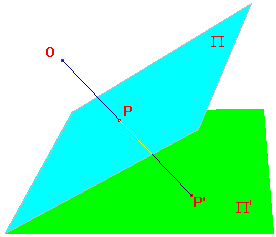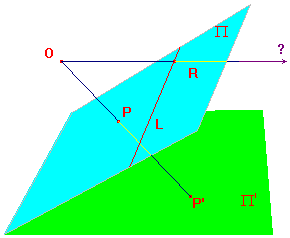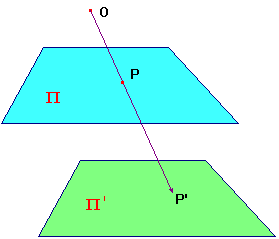Perspectivities - the geometric definition

In this section we give the geometric definition of a family of maps
whch generate the projective group P(2). We begin with a partial
map from one plane to another, and see that this extends naturally
to a bijecton between the corresponding extended planes.

 Preliminary Defintion If Π and Π' are planes in 3-dimensional space, and O is a point which lies on neither plane, then the perspectivity from Π to Π' with vertex O, is the map t which maps P on Π to P' on Π', the point where OP cuts Π' (if it exists). In our initial discussions, we consider only the cases where Π and Π' are non-parallel. These provide clear motivation for the extension to extended planes. The parallel case is easier, and is dealt with at the end. Shortly, we will see why the proviso is needed in general. Note that, whatever plane Π' is chosen, P' (if it exists) will lie on the line OP. Note also that, if we reverse the roles of Π and Π', then we get a map taking P' to P, i.e. t-1 is the perspectivity from Π' to Π with vertex O.Now suppose that P, Q on Π have images P', Q' on Π'. Let Π* denote the plane through O, P and Q. For any point R on the line PQ, the line OR will lie on Π*. It follows that the image of R (if it exists) will lie on the the line of intersection of Π* and Π'. In this case, the line PQ maps to a line on Π'. We assumed that the line PQ was such that the plane Π* met Π'. Of course, for some choices of P and Q, we will have Π* = OPQ parallel to Π'. This will happen precisely when P and Q lie on the line L where Π meets the plane through O parallel to Π'. This is discussed in the next pargraph.In this picture, L is on Π and parallel to Π'. For P not on L, OP is not parallel to Π', so meets Π'. For R on L, OR is parallel to Π', so t(R) cannot be defined as the intersection of OR and Π'. To see how we can define t(R), we first consider the family of lines on Π passing through R other than L. Since each such line is not parallel to Π', its image is a line on Π'. Since two such lines meet only in R, and R has no image, the images do not meet on Π'. Thus, the images are a family of parallel lines on Π'. When defining extended planes, we defined an ideal point for Π' as a new "point" added to each member of a family of parallel lines. Thus, we say that t(R) is the ideal point of Π' defined by the family of images. Since each point of L maps to an ideal point of Π', this means that the image of L itself is the ideal line of Π'.Finally, by considering the perspectivity t-1, we see that there is a line L' on Π' mapped by t-1 to the ideal line of Π. It follows that the ideal line of Π should have image L'. We now have a bijection from the extended plane Π to the extended plane Π'. We still denote it by t, and refer to it as a perspectivity. Now suppose that Π and Π' are parallel as shown. Then for any P on Π, OP meets Π', so t(P) is always defined as a point of Π', and each point of Π' arises in this way. The image of a family of parallel lines on Π will be a family of lines on Π'. The latter family will also be parallel, since any intersection would be the image of a point on Π, and this would lie on the original family. Thus t may be said to map the ideal points of Π to those of Π'. Again we have a map of the extended planes. In fact, the perspectivities generate P(2), as we shall see.